Splitting Of D Orbital Energies In Fields Of Other Symmetry - Coordination Chemistry

# Splitting Of D Orbital Energies In Fields Of Other Symmetry - Coordination Chemistry - Inorganic Chemistry

Splitting of d Orbital Energies in Fields of Other Symmetry

Tetrahedral Complexes: In tetrahedral complexes, none of the ligands approach directly any of the d orbitals. However, the ligands come closer to t he orbitals directed to edges of the cube (i.e. dxy, dyz and dzx) than those directed to the centres of the cube (dx2-y2 and dz2).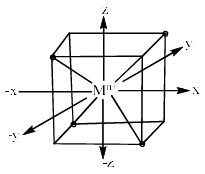Therefore, the dxy, dyz and dzx orbitals of metal cation experience more repulsion and of higher energy than t hose of dx2 – y2 and dz2 orbitals. After splitting, the dxy. dyz and dzx destabilize (t2 set) b y a factor of 0.4 ∆t and the dx2 – y2 and dz2 orbitals (e set) are stabilized by 0.6 ∆t. The difference in energy of t2 and e set is denoted as ∆t where t stands for tetrahedral. The crystal field splitting in tetrahedral complexes is shown below.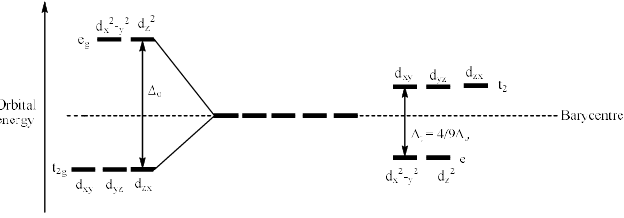Since ∆t is significantly smaller than ∆o, tetrahedral complexes are high-spin. Also, since smaller amounts of energy are needed for a t2 to e transition (tetrahedral) than for an eg to t2g transition (octahedral), corresponding octahedral and tetrahedral complexes often have different colours.

Tetrahedral complexes are always high spin because:
(a) ∆t = 4/9 ∆0 i.e. ∆t is much smaller than ∆0.
(b) ∆t is alwa ys much smaller than the pairing energy.

Due to these reasons, no pairing occurs in d3, d4, d5, d6, and d7 tetrahedral complexes. Therefore, tetrahedral complexes are always high spin irrespective of strong or weak ligands.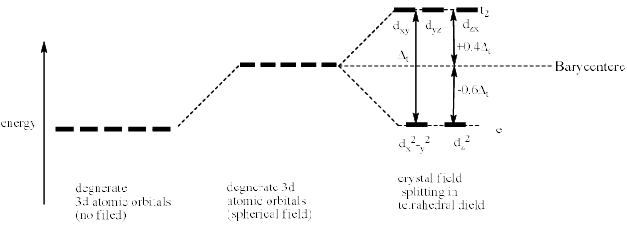The crystal field splitting in tetrahedral complexes is smaller than that in octahedral complexes. It is observed that
∆t = 4/9 ∆0

(a) In octahedral there are six ligands whereas in tetrahedral low ligands come to interact with metal ions. Hence the repulsion decreases by a factor of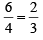(b) In octahedral, all the six ligands come along the axes while in tetrahedral they come between the axes. Therefore, again repulsion is decreases by a factor ofTo these two factors, the crystal field splitting decreases I tetrahedral by a factor of 4/9 than in octahedral i.e.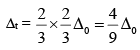CFSE in Tetrahedral Complexes

In tetrahedral CFSE = (no. of es in e orbital) × (–0.6 ∆t) + (no. of e-s in torbital) × (–0.4 ∆t)
or     CFSE = ne × (–0.6 ∆t) + nt2 × (+0.4∆t)

In terms of ∆o
CFSE = [ne × (–0.6) + nt2 × (+0.4 )] 4/9 ∆o
CFSE = [–0.27∆o × ne + 0.18∆o × nt2]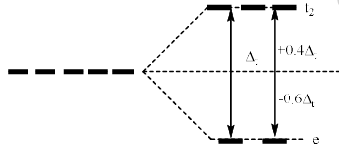The CFSE values for various configurations of tetrahedral complexes are given in the following table.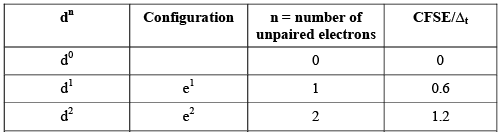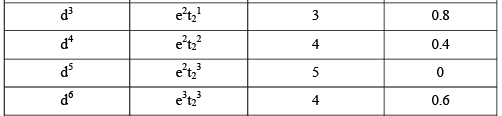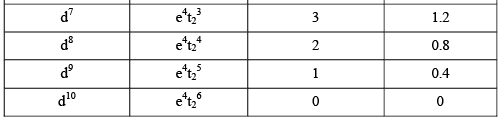Square Planar Complexes

A square planar arrangement of ligands can be formally derived from an octahedral array by removal of two trans ligands.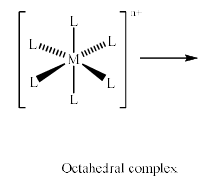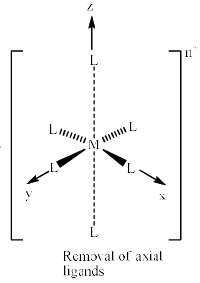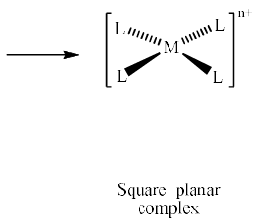If we remove the ligands lying along the z axis, then the dz2 orbital is greatly stabilized; the energies of the dyz and dxz orbitals are also lowered, although to a smaller extent. The resultant ordering of the metal d orbitals is shown below: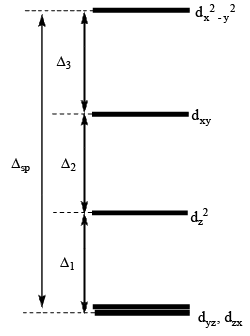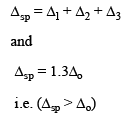The fact that square planar d8 complexes such as [Ni(CN)4]2– are diamagnetic is a consequence of the relatively large energy difference between the dxy and dx2 – y2 orbitals.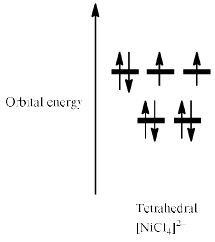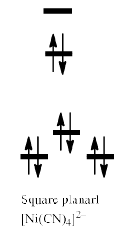Thus, [NiCl4]2– is paramagnetic while [Ni(CN)4]2– is diamagnetic. Alt hough [NiCl4]2– is tetrahedral and paramagnetic, [PdCl4]2– and [PtCl4]2– are square planar and diamagnetic. This difference is a consequence of the larger crystal field splitting observed for second and third row metal ions compared with their first row congener; Pd(II) and Pt(II) complexes are invariably square planar.

Other Crystal Fields

Crystal field splittings for some common geometries with the relative splittings of the d orbitals with respect to ∆o is given below. But this comparison is only valid for MLx type complexes containing like ligands, and so only applies to simple complexes.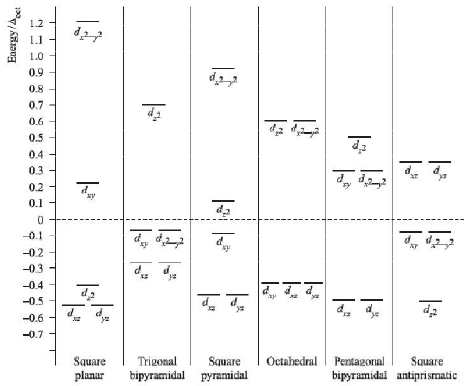The document Splitting Of D Orbital Energies In Fields Of Other Symmetry - Coordination Chemistry | Inorganic Chemistry is a part of the Chemistry Course Inorganic Chemistry.
All you need of Chemistry at this link: Chemistry

## FAQs on Splitting Of D Orbital Energies In Fields Of Other Symmetry - Coordination Chemistry - Inorganic Chemistry

 1. What is the splitting of d orbital energies in fields of other symmetry?Ans. The splitting of d orbital energies in fields of other symmetry refers to the phenomenon where the energies of the d orbitals in a coordination complex are split into different levels due to the presence of a ligand field. This splitting occurs because the ligands surrounding the central metal ion generate an electric field that interacts with the d orbitals, causing them to have different energies.
 2. How does the symmetry of the ligand field affect the splitting of d orbital energies?Ans. The symmetry of the ligand field plays a crucial role in determining the splitting of d orbital energies. In general, ligand fields with lower symmetry (e.g., tetrahedral or trigonal pyramidal) result in a smaller splitting of the d orbitals, while ligand fields with higher symmetry (e.g., octahedral) lead to a larger splitting. This is because lower symmetry fields allow for less effective overlap between the d orbitals and the ligands, resulting in a smaller energy difference between the split levels.
 3. How can the splitting of d orbital energies be experimentally determined?Ans. The splitting of d orbital energies can be experimentally determined through various spectroscopic techniques. One commonly used method is electronic absorption spectroscopy, where the absorption of light by the coordination complex is measured. The energy difference between the absorbed light and the ground state of the complex corresponds to the energy splitting of the d orbitals. Another technique is magnetic susceptibility measurement, which involves measuring the response of the complex to an external magnetic field. The magnitude of the splitting can be inferred from the magnetic properties of the complex.
 4. What factors influence the magnitude of the splitting of d orbital energies?Ans. Several factors influence the magnitude of the splitting of d orbital energies. These include the nature of the metal ion, the identity and coordination of the ligands, and the overall symmetry of the complex. For example, transition metal ions with higher charges tend to have larger energy splittings. Similarly, ligands with strong electron-donating or electron-withdrawing abilities can affect the splitting. Additionally, the geometry of the coordination complex, such as octahedral or tetrahedral, also influences the magnitude of the splitting.
 5. How does the splitting of d orbital energies affect the properties of coordination complexes?Ans. The splitting of d orbital energies has significant implications for the properties of coordination complexes. It determines the color of many coordination compounds as it is responsible for the absorption and reflection of light. The splitting also affects the magnetic properties of the complexes, such as their paramagnetic or diamagnetic behavior. Furthermore, the energy difference between the split levels influences the reactivity and stability of the complexes, particularly in terms of their ability to undergo ligand exchange reactions or catalytic processes.

## Inorganic Chemistry

40 videos|90 docs|37 tests

## Inorganic Chemistry

40 videos|90 docs|37 tests
Signup to see your scores go up within 7 days! Learn & Practice with 1000+ FREE Notes, Videos & Tests.
10M+ students study on EduRev
Track your progress, build streaks, highlight & save important lessons and more!(Scan QR code)
Related Searches

,

,

,

,

,

,

,

,

,

,

,

,

,

,

,

,

,

,

,

,

,

;